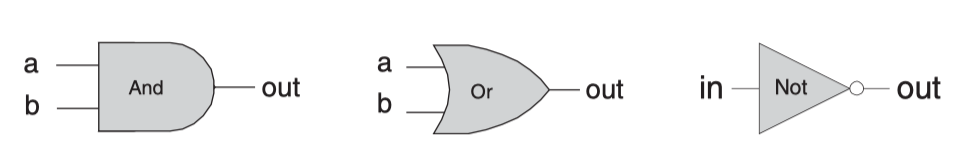# Nand2tetris - 布尔逻辑

• 布尔逻辑的基本操作可以只有一个：nand
• and, or, not 等操作都能以 nand 的组合来表达。
• 任何01串到01串的映射，都能以表格（真值表）的形式表达，我们能将真值表翻译成由 and, or, not 组成的逻辑表达式。CHIP Not {
IN in;
OUT out;

PARTS:
Nand(a=in, b=in, out=out);
}
CHIP And {
IN a, b;
OUT out;

PARTS:
Nand(a=a, b=b, out=outTmp);
Not(in=outTmp, out=out);
}
CHIP Or {
IN a, b;
OUT out;

PARTS:
Not(in=a, out=notA);
Not(in=b, out=notB);
Nand(a=notA, b=notB, out=out);
}
CHIP Xor {
IN a, b;
OUT out;

PARTS:
Or(a=a, b=b, out=orAb);
Nand(a=a, b=b, out=not11);
And(a=orAb, b=not11, out=out);
}
CHIP Mux {
IN a, b, sel;
OUT out;

PARTS:
Not(in=sel, out=notSel);
// sel = 0, result = a, else resut = 1
Or(a=a, b=sel, out=selA);
// sel = 1, result = b else resut = 1
Or(a=b, b=notSel, out=selB);
And(a=selA, b=selB, out=out);
}
CHIP DMux {
IN in, sel;
OUT a, b;

PARTS:
Not(in=sel, out=notSel);
And(a=in, b=notSel, out=a);
And(a=in, b=sel, out=b);
}